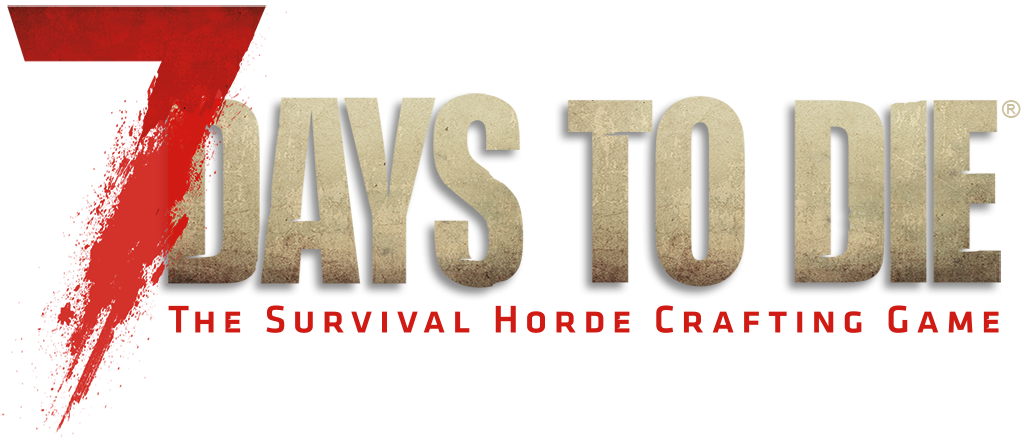Jump to contentbbh_blocked_dnftl

## Recommended Posts

Hey guys,

I wanna know if it is possible to mod the game so it increase harvesting, gathering and xp multipliers just like other survival games like ARK?

##### Share on other sites

Yeah you can rebalance everything but it will require a lot of work.

for eg.

progression.xml you can affect how much you harvest from skills / perks using the HarvestCount code

```<perk name="Miner 69er" icon="mining" description_key="miner69erDesc" title_key="miner69er" max_level="5" skill_point_cost_multiplier="1.43" skill_point_cost_per_level="5" group="tools">
<requirement perk_level="1" required_skill_name="Mining Tools" required_skill_level="1"/>
<requirement perk_level="2" required_skill_name="Mining Tools" required_skill_level="10"/>
<requirement perk_level="3" required_skill_name="Mining Tools" required_skill_level="20"/>
<requirement perk_level="4" required_skill_name="Mining Tools" required_skill_level="40"/>
<requirement perk_level="5" required_skill_name="Mining Tools" required_skill_level="80"/>
<effect name="BlockDamage">
<multiply perk_level="0" value="1"/>
<multiply perk_level="1" value="1.1"/>
<multiply perk_level="2" value="1.2"/>
<multiply perk_level="3" value="1.4"/>
<multiply perk_level="4" value="1.6"/>
<multiply perk_level="5" value="2.0"/>
</effect>
<multiply perk_level="0" value="1"/>
<multiply perk_level="1" value="0.9"/>
<multiply perk_level="2" value="0.8"/>
<multiply perk_level="3" value="0.7"/>
<multiply perk_level="4" value="0.6"/>
<multiply perk_level="5" value="0.5"/>
</effect>
[color="#FFFF00"]<effect name="HarvestCount">[/color]
<multiply perk_level="0" value="1"/>
<multiply perk_level="1" value="1.00"/>
<multiply perk_level="2" value="1.00"/>
<multiply perk_level="3" value="1.10"/>
<multiply perk_level="4" value="1.25"/>
<multiply perk_level="5" value="1.50"/>
</effect>
</perk>```

or you can can change how much each individual tool will gather specific resources, for eg. the fire axe will gather 50% of specified resources that are harvested using the butcher event, and 100% of resources that are harvested using the harvestingTools event

whereas the steel fire axe will will gather 75% of specified resources that are harvested using the butcher event, and 140% of resources that are harvested using the harvestingTools event

Items.xml

```<item id="107" name="fireaxeIron">
<property name="Meshfile" value="Items/Weapons/Melee/Axe/fireaxePrefab"/>
<property name="Material" value="metal"/>
<property name="RepairTools" value="forgedIron"/>
<property name="HoldType" value="17"/>
<property name="SoundJammed" value="ItemNeedsRepair"/>
<property name="FuelValue" value="30"/>
<property name="EconomicValue" value="240"/>
<property name="SoundDestroy" value="wooddestroy1"/>
<property class="Action0">
<property name="Class" value="Melee"/>
<property name="Delay" value="1.1"/>
<property name="Range" value="2.1"/>
<property name="Sphere" value="0.1"/>
<property name="Block_range" value="4"/>
<property name="Sound_start" value="swoosh"/>
<property name="Stamina_usage" value="8.27"/>
<property name="DamageBonus.wood" value="1"/>
<property name="DamageBonus.glass" value="0.25"/>
<property name="DamageBonus.stone" value="0.25"/>
<property name="DamageBonus.metal" value="0.25"/>
<property name="DamageBonus.earth" value="0.2"/>
<property name="DamageBonus.organic" value="1"/>
<property name="ActionExp" value="4"/>
[color="#FFFF00"]<property name="ToolCategory.Butcher" value="0.5" param1="10"/>
<property name="ToolCategory.harvestingTools" value="1" param1="1"/>[/color]
<property name="Sound_harvesting" value="open_animal" param1="organic" />
</property>
<property class="Attributes">
<property name="EntityDamage" value="6,15"/>
<property name="BlockDamage" value="30,48"/>
<property name="DismembermentBaseChance" value="0,0.05"/>
</property>
<property name="Group" value="Tools/Traps"/>
<property name="ActionSkillGroup" value="Mining Tools"/>
<property name="CraftingSkillGroup" value="craftSkillTools"/>
<property name="RepairExpMultiplier" value="5.5"/>
</item>

<item id="108" name="fireaxeSteel">
<property name="Extends" value="fireaxeIron"/>
<property name="CustomIcon" value="fireaxeIron"/> <!-- xxx model to be replaced -->
<property name="CustomIconTint" value="a0a0ff"/>
<property name="Meshfile" value="Items/Weapons/Melee/Axe/fireaxePrefab"/>
<property name="RepairTools" value="forgedSteel"/>
<property name="EconomicValue" value="912"/>
<property class="Action0">
<property name="Stamina_usage" value="15"/>
[color="#FFFF00"]<property name="ToolCategory.Butcher" value="0.75" param1="10"/>
<property name="ToolCategory.harvestingTools" value="1.4" param1="1"/>[/color]
</property>
<property class="Attributes">
<property name="EntityDamage" value="9,22"/>
<property name="BlockDamage" value="30,72"/>
</property>
</item>```

in blocks.xml you can find the different harvest events for specific resources that are applied to each tool

```<block id="1" name="stone">
<property name="Material" value="stone"/>
<property name="Shape" value="Terrain"/>
<property name="Mesh" value="terrain"/>
<property name="Texture" value="1"/>
<property name="ImposterExchange" value="imposterBlock" param1="97"/>
<property name="DropScale" value="2"/>
<property name="LPHardnessScale" value="2"/>
<property name="Weight" value="125"/>
<property name="Map.Color" value="100,100,100"/>
<property class="RepairItems"> <property name="cobblestones" value="6"/> </property>
<property name="HarvestOverdamage" value="true"/> <!-- default=true -->
[color="#FFFF00"]<drop event="Harvest" name="rockSmall" count="25" tool_category="harvestingTools"/>
<drop event="Harvest" name="ironFragment" count="2" tool_category="harvestingTools"/>[/color]
<drop event="Destroy" count="0"/>
<drop event="Fall" name="rockSmall" count="50" prob="0.23" stick_chance="0"/>
<drop event="Fall" name="ironFragment" count="4" prob="0.23" stick_chance="0"/>
<drop event="Fall" name="destroyedStone" count="1" prob="0.1" stick_chance="0.5"/>
<!-- Better to have fewer stacks with higher numbers for performance reasons. -->
<!-- With a stick chance fallen resources just get buried and fall off the earth. -->
<!-- Could maybe do "0.1" stick but it would quickly make the whole endeavor unfun. Gazz -->
<property name="CanMobsSpawnOn" value="true"/>
<property name="EconomicValue" value="5"/>
<property name="EconomicBundleSize" value="1"/>
</block>```

Butcher events are found in the Entityclasses.xml for each animal

- - - Updated - - -

If you want to affect how much XP you get from using specific tools that is found in the <property name="ActionExp" value="4"/> in items.xml

which is how much XP you get for each contacted swing, and in the progression.xml each skill has a specified amount of XP required to gain per level

for eg. the XP for the first player level is 9545 and for level 2 you mulitply that by the XP multiplier and is 9687 and for level 3 it requires 9832 etc.

```<player
max_level="200"
exp_to_level="9545"
experience_multiplier="1.0149"
skill_points_per_level="6"
skill_point_multiplier="0"/>```

and for skills the default XP required for level 1 is 250 with a multiplier of 1.03, you can specify how much XP each skill requires directly within each skill

```<skills
max_level="100"
exp_to_level="250"
experience_multiplier="1.03"
skill_point_cost_multiplier="0"
skill_point_cost_per_level="1" >```

##### Share on other sites

thank you

@Clockwork Orange

is it possible to just put a multipliers on all resources instead of editing everything tool? like having say x3 on everything and every tool. so a swing from level 100 axe yelled say 2 resources, a level 600 yelled 10. can i just multiplier it so it x3 both of them without having to change everything for each tool.

##### Share on other sites

No the resources are gathered through the harvest events, you can either change the resources on each and every block and or the multipliers on the tools themselves.

Keep in mind that the amount of block damage the tools do will also affect how much resources are gathered as well

```<item id="108" name="fireaxeSteel">
<property name="Extends" value="fireaxeIron"/>
<property name="CustomIcon" value="fireaxeIron"/> <!-- xxx model to be replaced -->
<property name="CustomIconTint" value="a0a0ff"/>
<property name="Meshfile" value="Items/Weapons/Melee/Axe/fireaxePrefab"/>
<property name="RepairTools" value="forgedSteel"/>
<property name="EconomicValue" value="912"/>
<property class="Action0">
<property name="Stamina_usage" value="15"/>
<property name="ToolCategory.Butcher" value="0.75" [color="#FFFF00"]param1="10"[/color]/>
<property name="ToolCategory.harvestingTools" value="1.4" [color="#FFFF00"]param1="1"[/color]/>
</property>
<property class="Attributes">
<property name="EntityDamage" value="9,22"/>
[color="#FFFF00"]<property name="BlockDamage" value="30,72"/>[/color]
</property>
</item>```

The param1 code will multiply how much damage the tool does to specific resources as well along with the damage bonus code <property name="DamageBonus.wood" value="1"/>

#### Archived

This topic is now archived and is closed to further replies.

×

• #### Support

• Discord
• Guilded
×
• Create New...